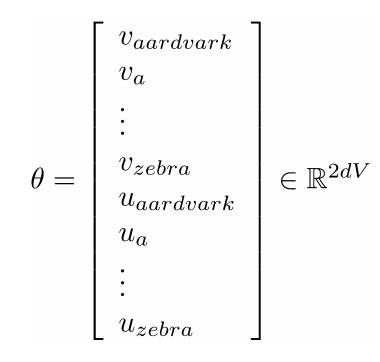Time Estimate

# Build a Model with Word2Vec

## Learning Objectives

After completing this unit, you’ll be able to:
• Explain what a vector gradient is.

We have the linguistics. We have the math. What’s next on our NLP journey?

## Training a Model

Now that you know the objective function, it’s time to train the model. Let’s start by reviewing the objective function.Remember that theta in the objective function stands for all the vectors in the vocabulary. This includes both the vectors for each word as the center word and the vectors for each word as the context word. For a simple vocabulary, you’d have some center word vectors (like vaardvark and vzebra), and some context word vectors (like uaardvark and uzebra).To train the model, we need to calculate the vector gradients for every vector in theta.

What’s a vector gradient? It’s the derivative, or rate of change, of a vector. This rate is what gives the machine a way to map every instance of a word and find the most appropriate vector. Thankfully, Word2vec calculates all the gradients for you!

It’s important to know how vector gradients work, however, as they come into play when we’re training our model.## Optimize Parameters with Gradient Descent

To optimize your model, you need to minimize the objective function J(θ). In other words, you want your model to have the smallest amount of error possible. One way to minimize J(θ) is an algorithm called gradient descent.

Here’s the basic idea: for the current value of theta (the current word vectors), calculate the gradient of J(θ). Then use that gradient information to take a small step in the direction of negative gradient by changing theta. Repeat these steps over and over, until you reach the minimum value for J(θ) (when the gradient of theta is zero).#### Note

In practice, J(θ) is not a simple convex function like this. Gradient descent is usually messier than this example, but always has the same goal of finding the lowest point on the function.

Gradient descent makes a lot of sense. However, by itself, it is an extremely large function. Remember, J(θ) includes all the windows in the input text, which could be billions!

This means that computing the gradient of J(θ) is very expensive. You would have to wait a very long time to make each update, as you calculate J(θ) over and over again.

How can you mitigate that problem? The answer is stochastic gradient descent. Rather than calculating the gradient over the entire body of text, you sample a single window (a single position in the text) at random and minimize the gradient based on that single window. Then you update the vectors for the words in the window you sampled. You repeat that process using many windows across the text, updating each time. Stochastic gradient descent can be very noisy, but it is much faster than traditional gradient descent, and over enough iterations, gives pretty good results.#### Note

In practice, you compromise between gradient descent and stochastic gradient descent. Rather than calculating the gradient over the entire dataset, or over a single window at a time, you calculate the gradient over mini-batches of data. Each mini-batch contains a few examples from the dataset (often 32, 64, 128, or 256 examples). You update your vectors after each mini-batch, repeating the process over many batches across the dataset.

## Other Approaches with Word2vec

Up to this point, this module has discussed predicting what context words you find around a specific center word. This approach is called the skip-gram model. Word2Vec also supports the continuous bag of words model. With the continuous bag of words model, rather than finding context words from a center word, you work to predict the center word, given context words.

There are lots of ways to train the model using Word2vec. In addition to the naive softmax method we have discussed in detail, Word2vec supports a technique called negative sampling. With negative sampling, rather than updating all the word vectors after each optimization step (as with gradient descent), or updating just the word vectors in your sample (as with stochastic gradient descent), you update both the word vectors in your sample and a set of negative words. This means that you identify a set of words that are very unlikely to be found near your center word (if you’re using the skip-gram model), and update their probability to zero. (If you’re using the continuous bag of words model, you identify a set of words very unlikely to be the center word, and update their probability to zero.)

## Get Hands On with Natural Language Processing

In this trail, you’ll complete problem sets using a Google product called Colaboratory. That means you have to have a Google account to complete the challenges. If you don’t have a Google account or you want to use a separate one, you can create an account here.

Once you have a Google account:
3. Go to Colaboratory.